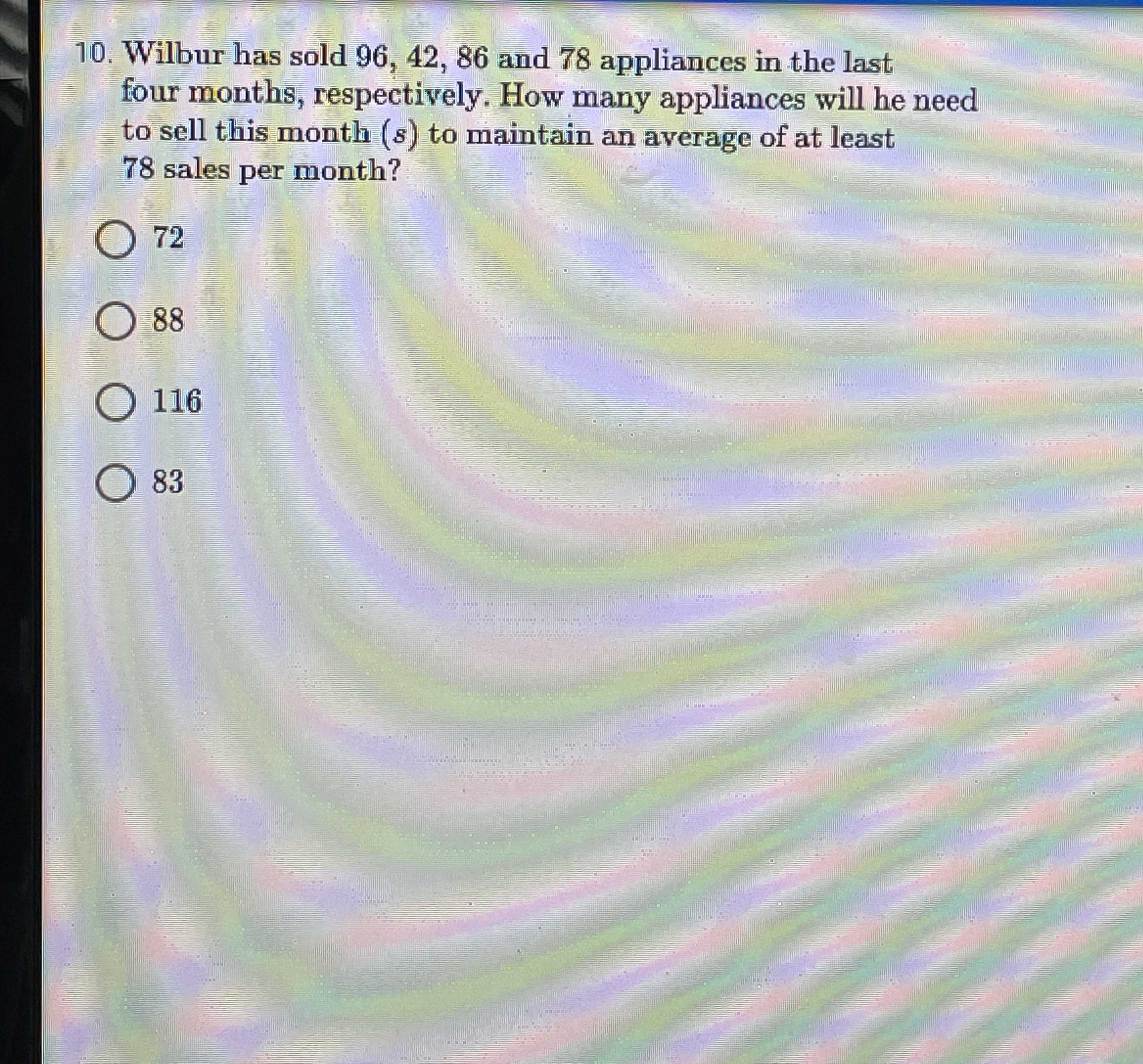### ¿Todavía tienes preguntas de matemáticas?

Pregunte a nuestros tutores expertos
Algebra
Pregunta10. Wilbur has sold $$96,42,86$$ and $$78$$ appliances in the last four months, respectively. How many appliances will he need to sell this month $$( s )$$ to maintain an average of at least

$$78$$ sales per month?

$$72$$

$$88$$

$$116$$

$$83$$ Wilbur has sold $$96,42,86$$ and $$78$$ appliances in the last four months, respectively. How many appliances will he need to sell this month ( $$s$$ ) to maintain an average of at least

$$78$$ sales per month?